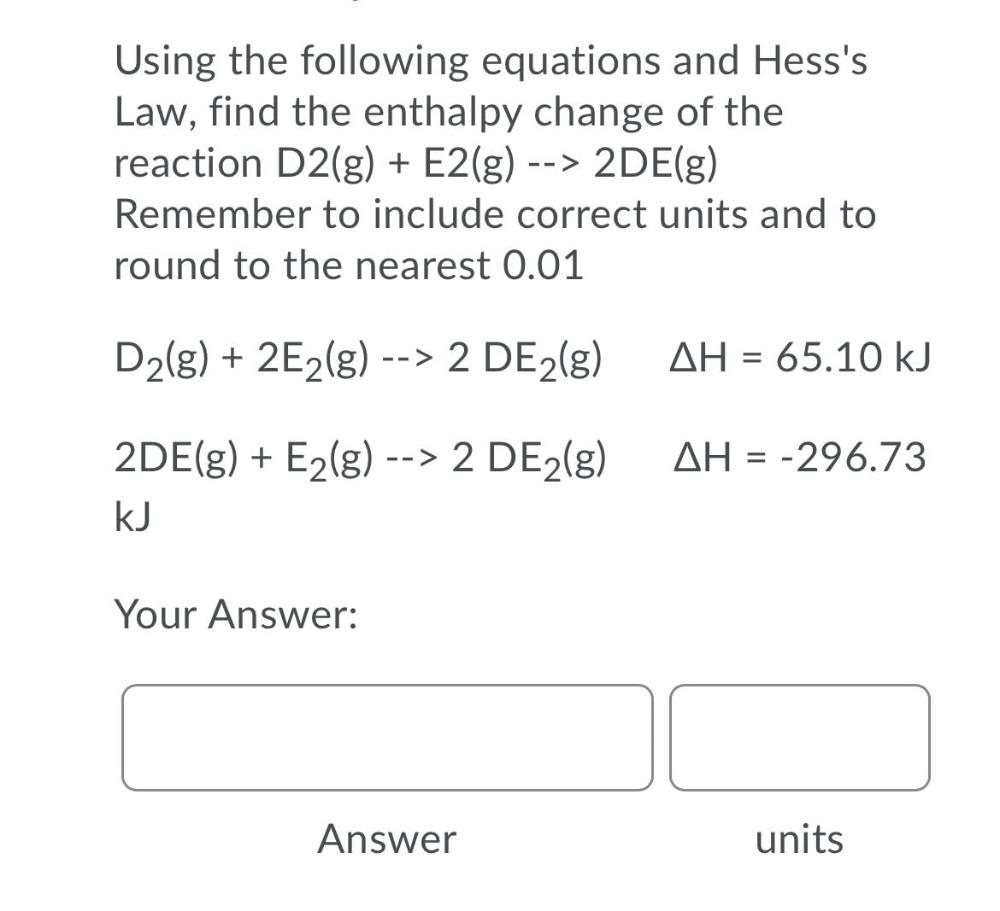Question:

# Using the following equations and Hess's Law, find theUsing the following equations and Hess's Law, find the enthalpy change of the reaction D₂(g) + E₂(g) --> 2DE(g) Remember to include correct units and to round to the nearest 0.01 D₂(g) + 2E₂(g) --> 2 DE₂(g) △H = 65.10 kJ 2DE(g) + E₂(g) --> 2 DE₂(g) △H = -296.73 kJ